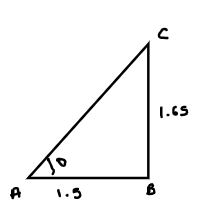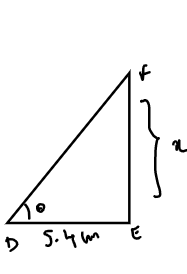Courses
Courses for Kids
Free study material
Free LIVE classes
MoreLIVE
Join Vedantu’s FREE Mastercalss

# A person $1.65m$ tall casts $1.5m$ shadow. At the same time, a lamp posts casts a shadow of $5.4m$.Find the height of lamp postVerified
360.6k+ views
Hint: Approach the solution by drawing the diagrams of a person and lamp posts with given data to know which concept is used.
Here a person is of $1.65m$ tall and cast shadow of $1.5m$
And also shadow of lamp post =$5.4m$
We know that the angle is the same as the incident that occurred at the same point in time.
So, here sum makes the same angle of hitting the top of the person and top of the lamppost.Therefore they are similar triangles $\Delta ABC\& \Delta DEF$
So, as they are the same triangles then $\Rightarrow \frac{{AB}}{{DE}} = \frac{{CB}}{{EF}}$their radius of side is also the same.
That means

$\Rightarrow \frac{{AB}}{{DE}} = \frac{{CB}}{{EF}}$
Here let $EF = xcm$
$\Rightarrow \frac{{1.5}}{{5.4}} = \frac{{1.65}}{x} \\ \Rightarrow x = \frac{{1.65 \times 5.4}}{{1.5}} \\ \Rightarrow x = 5.94m \\$
Therefore height of lamppost=$5.94m$

NOTE: In this type problems plotting the diagram with proper position gives the correct answer.Here we know that the angle is the same as the incident that occurred at the same point in time. So by using this condition on two diagrams we have equated the value and on further simplification we get the answer.

Last updated date: 19th Sep 2023
Total views: 360.6k
Views today: 10.60k